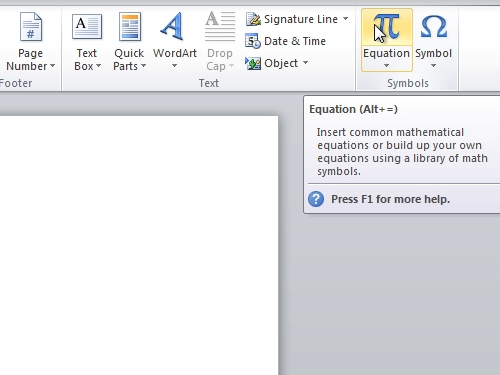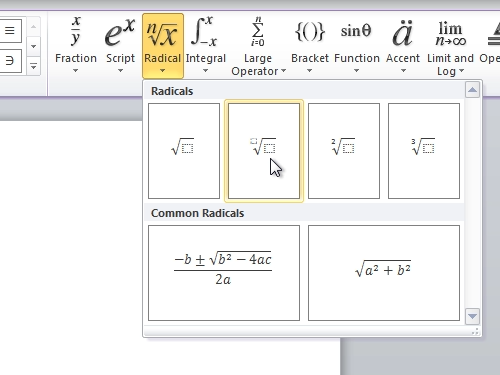## How to install Word 2010 Equation Editor

Learn how to open equation editor in Microsoft Word 2010

In this video tutorial we will show you how to install word 2010 equation editor.

In order to install word 2010 equation editor, open the Microsoft Word. Go to the “Insert” tab. Click on “Equation”.Now, you can type any formulas with the equation tools. For example, click on “Radical” and choose “Radicals” formula. Add data to your formula.Also, you can go to the “Insert” tab, then click on “equations” and choose various built-In formulas, such as “Binomial Theorem”.